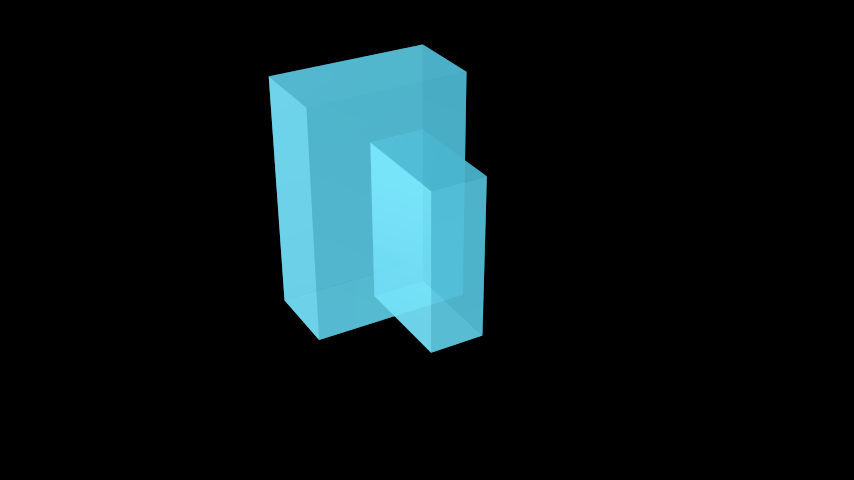# Prism#

Qualified name: `manim.mobject.three\_d.three\_dimensions.Prism`

class Prism(dimensions=[3, 2, 1], **kwargs)[source]#

Bases: `Cube`

A right rectangular prism (or rectangular cuboid). Defined by the length of each side in `[x, y, z]` format.

Parameters

dimensions (Sequence[int]) – Dimensions of the `Prism` in `[x, y, z]` format.

Examples

Example: ExamplePrism```from manim import *

class ExamplePrism(ThreeDScene):
def construct(self):
self.set_camera_orientation(phi=60 * DEGREES, theta=150 * DEGREES)
prismSmall = Prism(dimensions=[1, 2, 3]).rotate(PI / 2)
prismLarge = Prism(dimensions=[1.5, 3, 4.5]).move_to([2, 0, 0])
```

Methods

 `generate_points` Creates the sides of the `Prism`.

Attributes

 `animate` Used to animate the application of any method of `self`. `animation_overrides` `color` `depth` The depth of the mobject. `fill_color` If there are multiple colors (for gradient) this returns the first one `height` The height of the mobject. `n_points_per_curve` `sheen_factor` `stroke_color` `width` The width of the mobject.
generate_points()[source]#

Creates the sides of the `Prism`.

Return type

None